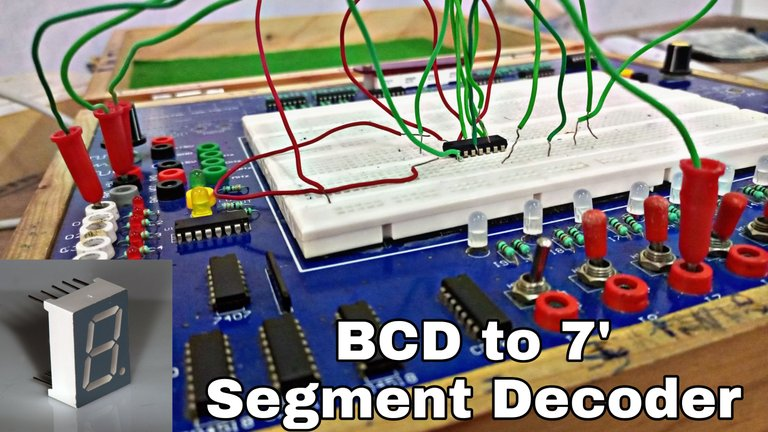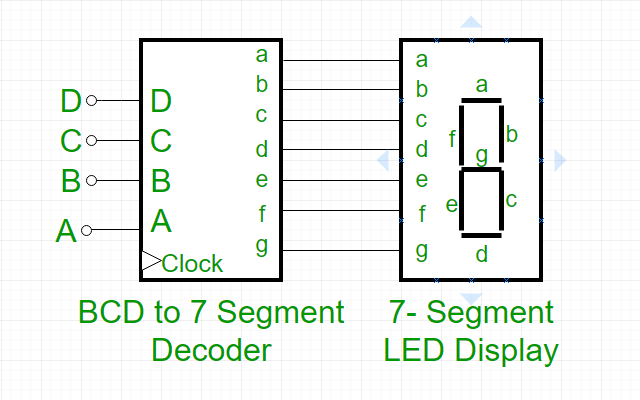# How does a Digital Watch work? (7 Segment Display Decoder)(Edited)

## 7 Segment Display Decoder

Today, we will know how the digital watch works and how its display works, we will also learn in great detail about it, although it is made up of a combination of 7 LEDs, as we would get the correct number according to the combinations mentioned above. This is called Seven segment decoder
To control 7 LEDs, we must take at least 3 input binary digits, with the help of which we can print numbers up to 9.### Three Input Binary Number

We have been familiar with the decimal number since childhood and often know the same. Decimal numbers are numbers whose base is 10 and binary numbers are numbers whose base is 2. In decimal only 1 to 9. There is a combination of numbers whereas in binary there is only a combination of 0,1, meaning what is meant by pot combination is that we can make 7 segment decoder only by using 3 types of binary numbers and their combination.

There are 7 types of linear LED lights and by making a combination of these, we can see the number of decimals on the display and to understand it, it is a,b,c,d,e,f,g respectively in clockwise order. Let's keep it so that we can solve it.

### TABLE

Input AInput BInput CInput DabcdefgOutput
000011111100
000101100001
001010011012
001111110013
010001100114
010110110115
011010111116
011111100007
100011111118
100111110119

### Karnaugh Map (K-Map)

This periodic method helps to solve any boolean expression most easily, we can solve any boolean expression without boolean algebra, and in the Seven Segment decoder, we use 3*2 table a,b, To solve c,d,e,f,g separately.src

### Clock Pluse

Clock pulse In this, clock plus is done because it is not enabled without clock plus and clock plus is also edge triggered because when the level triggered it generates race round condition, we edge-triggered only to remove it. Let's do that so that this condition doesn't happen.

Posted with STEMGeeks

0
0
0.000